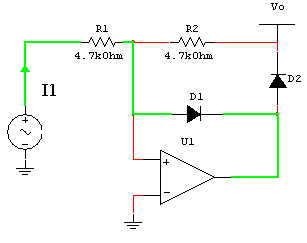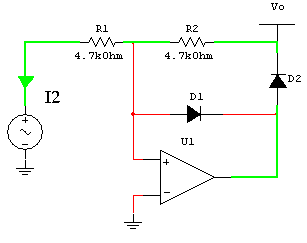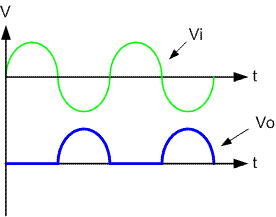# Electronic Device And Electronic Circuit

Data of electronic device , PCB Design and electronic circuitWeb basicelectronic.blogspot.com

## Tuesday, August 15, 2006

### Half-wave precision rectifiers circuit

A half-wave rectifier is a circuit that passes only the positive or only the negative
Portion of a wave ,while blocking out the other portion
Rectifiers are impremented using diodes. The nonzero forward-voltage drop of
A pactical diode may cause intolerable errors in low-level signal

Proceeding of half-wave precision rectifiers circuit we separate are two case
Case 1 Vi > 0 negative input of op amp is higher positive input ,the op amp
Output = 0 ,I1 will flow through R1 and D1 ,hence Vo = 0Case 2 Vi <>
Output = 1 ,I2 will flow through R2,R1 and D2 ,but the voltage at positive input
Must equal the voltage at negative input ,hence I2 = (0-Vi)/R1 = (Vo-0)/R2
This gives Vo = (-R2/R2)ViCircuit behavior
Vo = 0 for Vi > 0
Vo = -(R2/R1)Vi for Vi <>
Example waveform